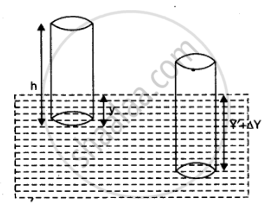CBSE (Science) Class 11CBSE
Share

# The Cylindrical Piece of the Cork of Density of Base Area A And Height H Floats in a Liquid of Density Rho_1. the Cork is Depressed Slightly and Then Released. Show that the Cork Oscillates up and Down Simple Harmonically with a Periodwhere ρ Is the Density of Cork. (Ignore Damping Due to Viscosity of the Liquid). - CBSE (Science) Class 11 - Physics

ConceptSome Systems Executing Simple Harmonic Motion

#### Question

The cylindrical piece of the cork of density of base area and height floats in a liquid of density rho_1. The cork is depressed slightly and then released. Show that the cork oscillates up and down simple harmonically with a period

T = 2pi sqrt((hrho)/(rho_1g)

where ρ is the density of cork. (Ignore damping due to viscosity of the liquid).

#### Solution 1

Base area of the cork = A

Height of the cork = h

Density of the liquid = rho_1

Density of the cork = ρ

In equilibrium:

Weight of the cork = Weight of the liquid displaced by the floating cork

Let the cork be depressed slightly by x. As a result, some extra water of a certain volume is displaced. Hence, an extra up-thrust acts upward and provides the restoring force to the cork.

Up-thrust = Restoring force, F = Weight of the extra water displaced

F = ­–(Volume × Density × g)

Volume = Area × Distance through which the cork is depressed

Volume = Ax

∴ F = – A x rho_1 g … (i)

According to the force law:

F = kx

k = F/x

Where, k is a constant

k = F/x = -Arho_1g  ...(ii)

The time period of the oscillations of the cork:

T = 2pi sqrt(m/k)   ...(iii)

Where

m = Mass of the cork

= Volume of the cork × Density

= Base area of the cork × Height of the cork × Density of the cork

Ahρ

Hence, the expression for the time period becomes:

T = 2pi sqrt((Ahrho)/(Arho_1g)) = 2 pi sqrt((hrho)/(rho_1g)

#### Solution 2

Say, initially in equilibrium, y  height of cylinder is inside the liquid. Then,

Weight of the cylinder = upthrust due to liquid displaced

:. Ahrhog = Ayrho_1g

When the cork cylinder is depressed slightly by triangle y and released, a restoring force, equal to additional upthrust, act on it. The restoring force is

F =  A(y + triangle y) rho_1 g - Ayrho_1g = Arho_1g triangle y

:. Acceleration, a = F/m = (Arho_1g triangle y)/(Ahrho) = (rho_1g)/(hrho). triangle y   and the acceleration is directed in a  direction opposite to triangle y. Obviously, as a prop - triangle y, the motion of cork cylinder is SHM, whose time period is given by

T = 2pi sqrt("displacement"/"accelertion")

=2pi sqrt((triangle y)/a)

= 2pi sqrt((hrho)/(rho_1g))Is there an error in this question or solution?

#### Video TutorialsVIEW ALL 

Solution The Cylindrical Piece of the Cork of Density of Base Area A And Height H Floats in a Liquid of Density Rho_1. the Cork is Depressed Slightly and Then Released. Show that the Cork Oscillates up and Down Simple Harmonically with a Periodwhere ρ Is the Density of Cork. (Ignore Damping Due to Viscosity of the Liquid). Concept: Some Systems Executing Simple Harmonic Motion.
S